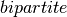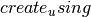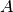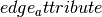Warning

This documents an unmaintained version of NetworkX. Please upgrade to a maintained version and see the current NetworkX documentation.

from_biadjacency_matrix(A, create_using=None, edge_attribute='weight')[source]

Creates a new bipartite graph from a biadjacency matrix given as a SciPy sparse matrix.

Parameters: A (scipy sparse matrix) – A biadjacency matrix representation of a graph create_using (NetworkX graph) – Use specified graph for result. The default is Graph() edge_attribute (string) – Name of edge attribute to store matrix numeric value. The data will have the same type as the matrix entry (int, float, (real,imag)).

Notes

The nodes are labeled with the attributeset to an integer 0 or 1 representing membership in part 0 or part 1 of the bipartite graph.

Ifis an instance of networkx.MultiGraph or networkx.MultiDiGraph and the entries ofare of type int, then this function returns a multigraph (of the same type as) with parallel edges. In this case,will be ignored.

biadjacency_matrix(), from_numpy_matrix()